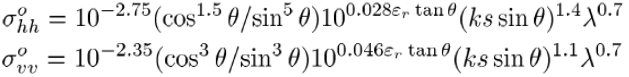# Incidence Angle for Soil Moisture

Dear Forum Members

I am trying to implement the Dubois and the Oh models for soil moisture and surface roughness estimation from Radarsat-2 data. I am referring to the following paper,
Mapping Soil Moisture Using RADARSAT-2 Data and Local Autocorrelation Statistics. Merzouki et al., IEEE JSTARS, 2011.
Here I notice that one needs the backscattering coefficients (I guess not in dB but the intensity Sigma0 after range doppler terrain correction (RDTC)) and the local incidence angles.

While performing the range doppler terrain correction of the Sigma0 images I see there are three options for generating the angles.

a) Incidence angle from ellipsoid
b) Local incidence angle
c) Projected local incidence angle

Can someone clarify which is the right angle to be used for this purpose and if I am right to think that the model has to be implemented on the Sigma0 obtained after the RDTC but not in the dB form?

I’d go for this one.

in chapter IV they talk of dB values, so I guess they have been used in the modelling instead of linear scale data.

Isn’t it counter intuitive to think that the model output will be in dB rather than in linear scale?

Why should it?
Data in dB scale has a gaussian-shaped histogram while linear scaled data has a gamma distribution. I’d prefer the first for most mathematical or statistical operations.

I mean when you feed in the values in the Dubois or Oh model the forward modelling outcome should be in the linear scale. Take for instance the Dubois model,I would think of using linear values of the backscattering coefficient on the LHS for inverting the roughness and relative dielectric constant on the RHS for a given local incidence angle and wave number.

Ok, you probably have spent more time with these equations, I just guessed from what I read in the article.